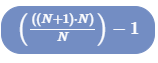# Simple Algorithm - I need clarity of Expression and Formula

#### NotGoodAtAll

##### New member
Hi,

Simple algorithm:

While n is greater than 1
Print n ! symbols
go to next line
make n equal n-1

If n - 5 the output reads:
!!!!!
!!!!
!!!
!!

There would be no single ! symbol as 1 is not greater than 1 (I assume this is correct, please correct me if not)

I need an expression that gives the number of symbols regardless of the value n, and write a formula.

I have drawn this out, bashed my head against the wall and I seem to be having an issue.

I have so far ascertained that the following works but not sure what else I can do:Any assistance would be hugely appreciated.

#### NotGoodAtAll

##### New member
I've also hit upon the following eqaution:

n = n-1 + n

where n-1 is the previous value of n in a recursive set. That make any better sense?

#### lev888

##### Full Member
You have a sequence n, n-1, ...,, 2. This is an arithmetic sequence. There is a formula for the sum of such sequence. Can you look it up?

#### JeffM

##### Elite Member
None of it makes much sense including the algorithm, which does not say what is to be done if n = 1. So how do you know that the last line prints an exclamation point?

In any case is the n! a symbol for n factorial?

What was the exact and complete statement of the problem?

#### NotGoodAtAll

##### New member
The full problem was presented as is:

Consider the following simple Algorithm:

While n is greater than 1
Print n ! symbols
go to next line
make n equal n-1

Show what is printed when n = 5

Then come up with an expression that would be true for the amount of ! marks regardless of the value of n. Write down the equation you could use.

My understanding is that the value for 1 doesn't exist, as 1 is not greater than 1 and the algorithm couldn't run in this case. The operative word "While" suggests a recursive algorithm until we reach 1 and then stop.

For background I'm working through a cryptography problem paper, nothing spectacular and strictly for recreation.

Hope this provides a little clarity.

#### NotGoodAtAll

##### New member
You have a sequence n, n-1, ...,, 2. This is an arithmetic sequence. There is a formula for the sum of such sequence. Can you look it up?
Hi, yes I have looked at it. the sequence I mean, and that's why i stumbled across the n=n-1 +n type of solution.

I'm trying to get my head around it, I don't much care for the Answer, more the solution, if you get my meaning? I want to understand how to come to the right expression and equation.

Cheers

#### lev888

##### Full Member
The full problem was presented as is:

Consider the following simple Algorithm:

While n is greater than 1
Print n ! symbols
go to next line
make n equal n-1

Show what is printed when n = 5

Then come up with an expression that would be true for the amount of ! marks regardless of the value of n. Write down the equation you could use.

My understanding is that the value for 1 doesn't exist, as 1 is not greater than 1 and the algorithm couldn't run in this case. The operative word "While" suggests a recursive algorithm until we reach 1 and then stop.

For background I'm working through a cryptography problem paper, nothing spectacular and strictly for recreation.

Hope this provides a little clarity.
For the formula see my post above. Regarding the algorithm, I would say "while" suggests a loop (iteration), not recursion.

#### lev888

##### Full Member
Hi, yes I have looked at it. the sequence I mean, and that's why i stumbled across the n=n-1 +n type of solution.

I'm trying to get my head around it, I don't much care for the Answer, more the solution, if you get my meaning? I want to understand how to come to the right expression and equation.

Cheers
You want to derive the formula yourself?

#### NotGoodAtAll

##### New member
So I have come to this:

General Expression
Xn= Xn-1 + n
X5= 9 + 5
X5= 14

Equation

((n+1)*n) / 2) - 1

I have somewhat buried myself in something else, so have been distracted but his is what I noted in my last scribbles. Can anyone advise if either one of these makes sense? before I go back to my notes and see why I got to this?

Many thanks

#### pka

##### Elite Member
So I have come to this:

General Expression
Xn= Xn-1 + n
X5= 9 + 5
X5= 14
Equation
((n+1)*n) / 2) - 1 CORRECT!
The sum of the first $$\displaystyle N$$ positive integers is $$\displaystyle \frac{N(N+1)}{2}$$. But you do not use 1 so subtract it off.

#### Jomo

##### Elite Member
...l as 1 is not greater than 1 (I assume this is correct, please correct me if not)
If 1 is greater than 1, then I have just one question for you. How much greater is 1 than 1?